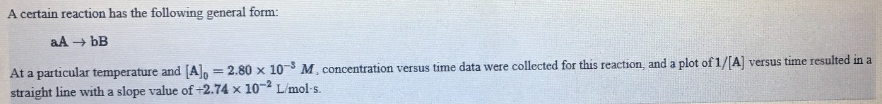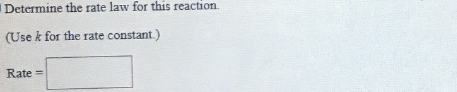# A certain reaction has the following general form: aA → bB At a particular temperature and [A]0 = 2.80 x 10^-3 M, concentration versus time data were collected for this reaction, and plot of 1[A] versus time resulted in a straight line with a slope value of -2.74 x 10^-2 L/mol.s. Determine the rate law for this reaction. (Use k for the rate constant)